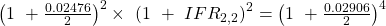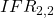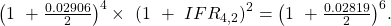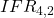Introduction to Fixed Income Valuation | IFT World
IFT Notes for Level I CFA® Program
IFT Notes for Level I CFA® Program

# Part 6

## 4.  The Maturity Structure of Interest Rates

### Maturity or Term Structure of Interest Rates

The term structure of interest rates is the relationship between interest rates and bonds with different times to maturity. The basic premise is that interest rates change when inflation rates are expected to change over a period of time. For example, a one-year zero-coupon bond may have an interest rate of 7.50%, while a two-year zero-coupon bond may have an interest rate of 9.75%, assuming all the other factors are the same (currency, credit rating, periodicity, etc.). The three common ways of representing the term structure of interest rates are given below:

Spot rate curve: In our example above, the interest rates of zero-coupon bonds, 7.50% and 9.75% are called spot rates. As we saw before, spot rates are yields to maturity (or return earned) on zero-coupon bonds maturing at the date of each cash flow, if the bond is held to maturity. So, the spot rate curve is also called the zero or strip curve. The spot rate curve plots different maturities on the x-axis and corresponding spot rates on the y-axis.

Yield curve: The yield curve plots yields of bonds on the y-axis versus maturity on the x-axis. The main difference between a yield curve and a spot rate curve is that the yield curve considers the coupon payments as well. The assumption in a spot rate curve is that there are no coupon payments.

Par curve: The par curve plots yields to maturity for different maturities, but the bonds are assumed to be priced at par.

### Forward Rates

A forward rate is an interest rate in the future. The one-year interest rate after one year is an example of a forward rate. The implied forward rate can be calculated using spot rates. As always, this is best understood with the help of an example. Consider a scenario where the one-year spot rate is 2%, and the two-year spot rate is 3%. This is illustrated below:

The forward rate f2 is the rate over the second year. This can be calculated if we assume the following: $1 invested for two years at the 2-year spot rate of 3% should give same result as$1 invested for 1 year at the one-year spot rate of 2% and then again at the forward rate, f2.  Mathematically, this can be expressed as: (1 + 0.03)2 = (1 + 0.02) (1 + f2)

Solving for f2, we get: f2 = 4.0098%.

The notation for a forward rate is expressed like this: 1y1y, 2y5y etc.; the first number refers to the length of the forward period in years from today and the second number refers to the tenor of the underlying bond. One of the applications of forward rates is that implied spot rates can be calculated as geometric averages of forward rates. Bonds can then be priced using implied spot rates. It gives the 1-year forward rate for zero-coupon bonds with various maturities. For example, 1y1y is the 1-year forward rate for a two-year bond.

 Time Period Forward Rate 0y1y 1.88% 1y1y 2.77% 2y1y 3.54% 3y1y 4.12%

Source: CFA Program Curriculum, Introduction to Fixed Income Valuation

Using the forward rates 0y1y and 1y1y, we can calculate the two-year spot rate as:

(1.0188) (1.0277) = (1 + z2)2

Calculating for z2, we get 2.32%.

A forward curve plots the forward rates, which is an estimation of what investors expect the short-term interest rates to be. Each rate on the curve has the same time frame. Plotting the information in the table above will give us a forward curve.

Example

 Maturity Price Yield to Maturity 1 year 97.25 2.476% 2 years 94.50 2.906% 3 years 91.30 2.819%

Compute the “1y1y” and “2y1y” implied forward rates stated on a semi-annual bond basis.

Solution:.= 0.0167 × 2 = 3.34%.

The 1y1y implied forward rate is 3.34%.= 0.0132 × 2 = 2.65%.

The 2y1y implied forward rate is 2.65%.